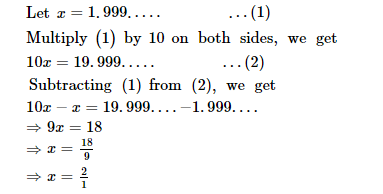# The value of 1.999. in the form of

Question:

The value of $1.999$. in the form of $\frac{m}{n}$, where $m$ and $n$ are integers and $n \neq 0$, is

Solution:Hence, the value of $1.999 \ldots$ in the form of $\frac{m}{n}$, where $m$ and $n$ are integers and $n \neq 0$, is $\frac{2}{1}$.